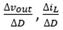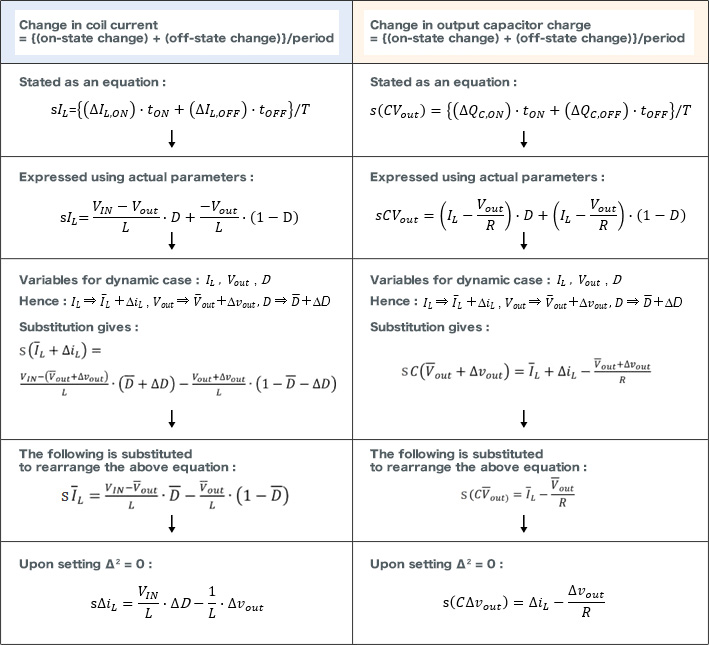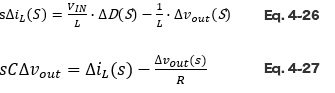# State-Space Averaging Method － Dynamic Analysis

2017.09.21

・The dynamic state quantities Δvout/ΔD and ΔIL/ΔDare derived from equations obtained by state averaging.

In the previous section, we performed a static analysis using equations derived by “state averaging”. This time, we will perform a dynamic analysis in order to derive, which is an objective of state averaging. Please read this in conjunction with the discussion of static analysis in the preceding section.

## Dynamic Analysis

Below are the previously derived equations relating to coil current and capacitor charge. They have appeared a number of times by now, but if the premises on which they are based are not kept in mind, solving the equations becomes difficult.

The same procedures are used with both equations, but here they are presented separately.Upon solving the last equations simultaneously,can be determined. The equations are rewritten as follows.Next time, we will consider the derivation of these equations from a somewhat different perspective.